# I got part a to be 1 and .92 1. Given the following matrix A- 05 .97 a. calculate the eigenvalues by using RStudio. b. calculate the integer values of the eigenvectors, vi and v2 by hand only calc...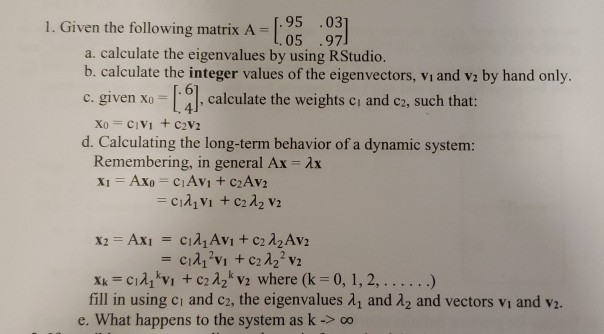I got part a to be 1 and .92

1. Given the following matrix A- 05 .97 a. calculate the eigenvalues by using RStudio. b. calculate the integer values of the eigenvectors, vi and v2 by hand only calculate the weights ci and c2, such that: c. given x d. Calculating the long-term behavior of a dynamic system: Remembering, in general Ax Ax fill in using ci and c2, the eigenvalues λ1 and λ2 and vectors vi and v2. e. What happens to the system as k -> oo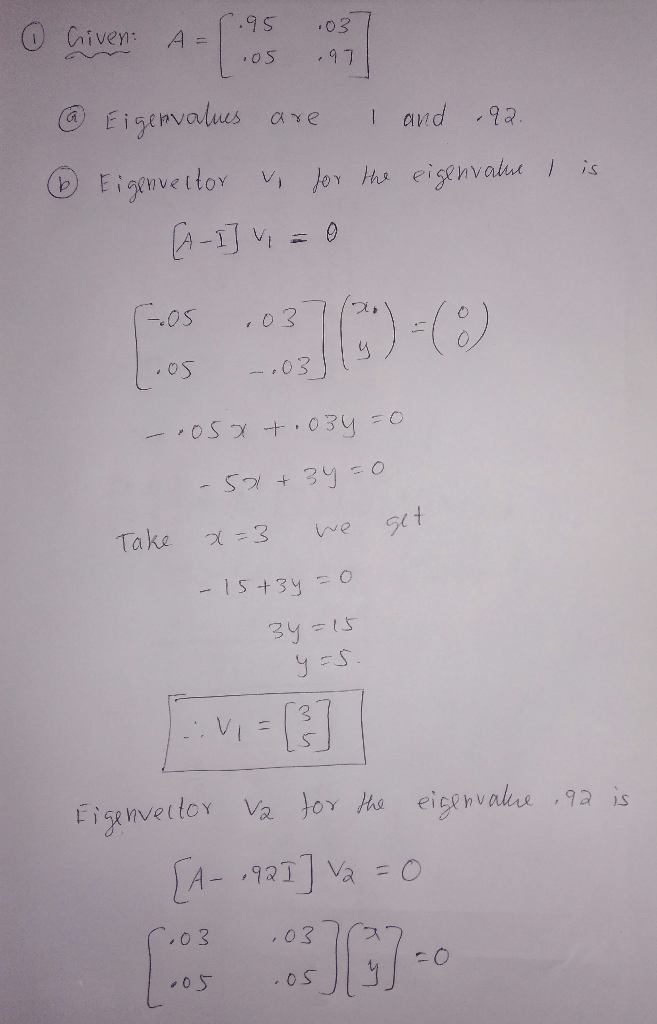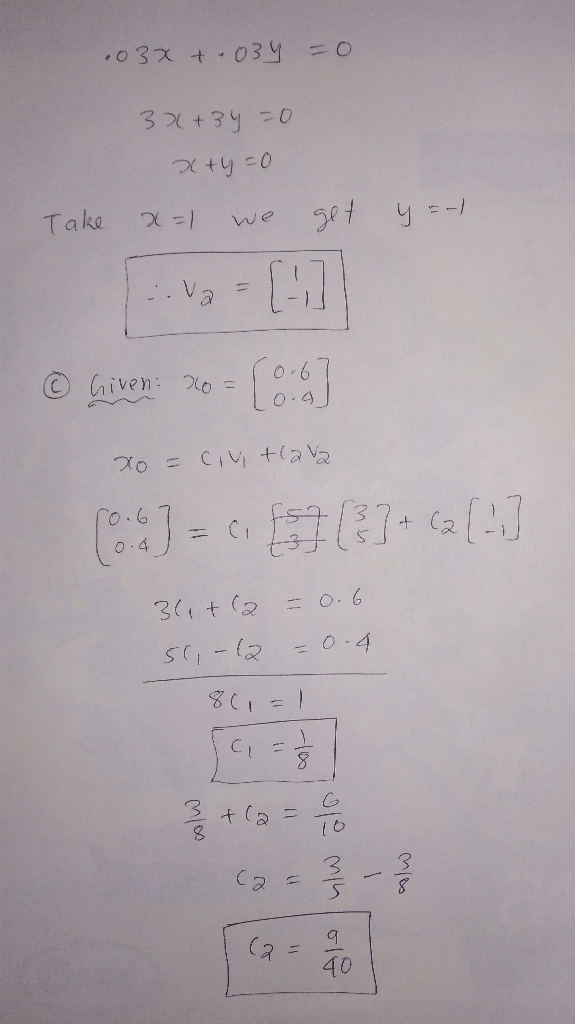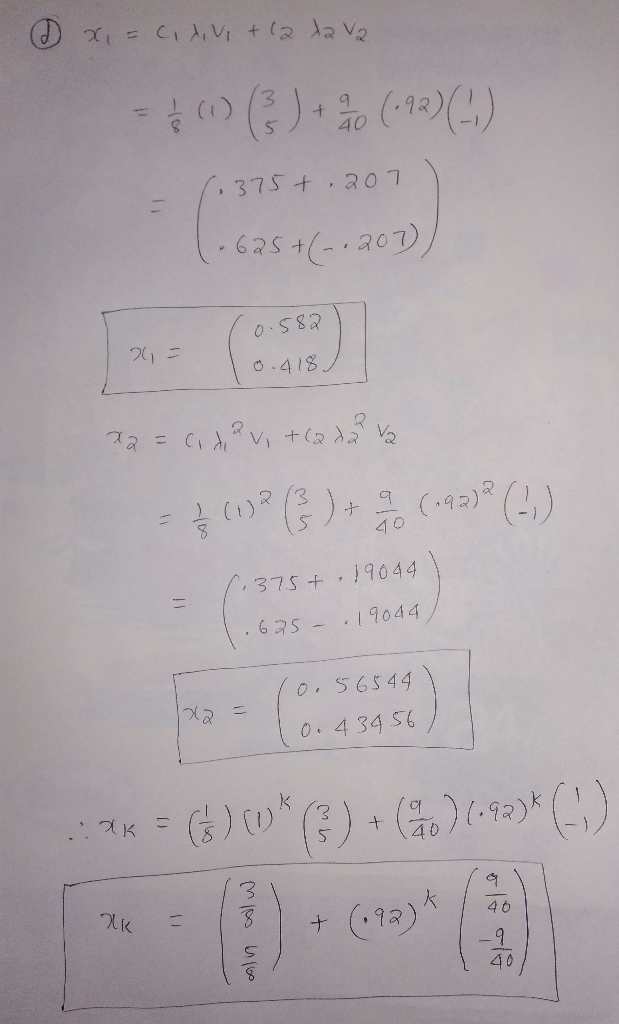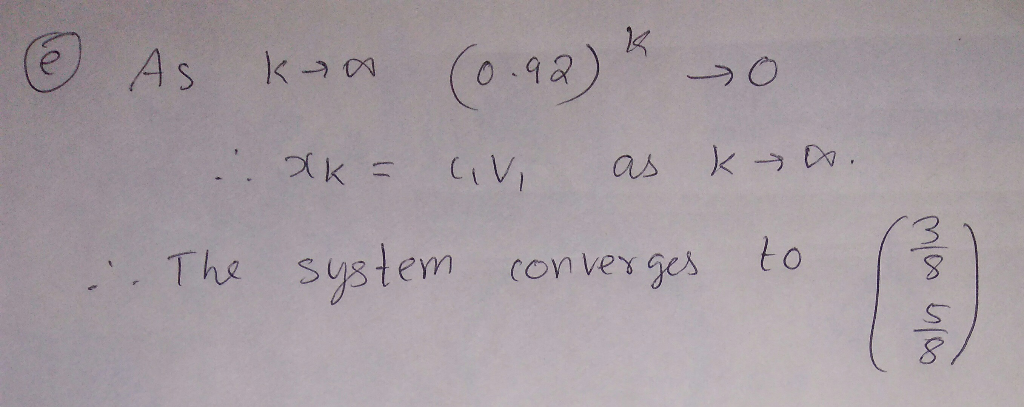##### Add Answer of: I got part a to be 1 and .92 1. Given the following matrix A- 05 .97 a. calculate the eigenvalues by using RStudio. b. calculate the integer values of the eigenvectors, vi and v2 by hand only calc...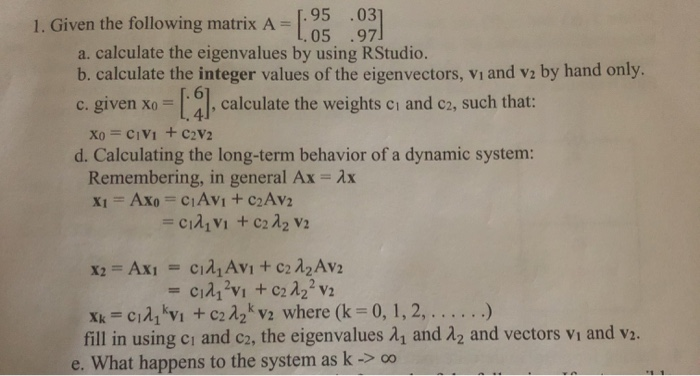.95 .03 L,05 .97 1. Given the following matrix A- a. calculate the eigenvalues by using RStudio. b. calculate the integer values of the eigenvectors, vi and vz by hand only. c. given Xoc d. Calculating the long-term behavior of a dynamic system: 6 4 , calculate the weights ci and c2, such that: Remembering, in general Ax Ax vezton viand s e. What happens to the system as k>o .95 .03 L,05 .97 1. Given the following matrix A-...